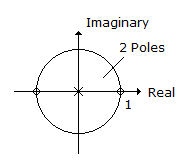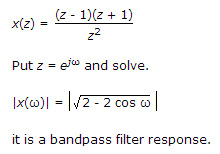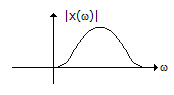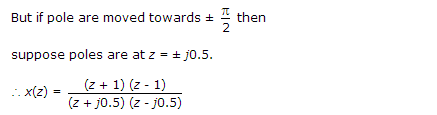# Electronics and Communication Engineering - Signals and Systems - Discussion

4.

Consider Pole zero diagram as shown, If two Poles are moved in opposite direction towards ω = p/2 and -p/2, the filter will be[A]. change to high pass filter [B]. change to Band Pass filter [C]. remains same [D]. change to LPF

Explanation:But still we will get the same response, zero are at same position.

 Nabil said: (Nov 15, 2014) I didn't understand the logic. Kindly provide me the detailed solution with proper reasoning.

 Susee said: (Feb 10, 2015) I cannot understood. Could you please explain me once?

 Brc said: (Mar 6, 2015) Can I take z=s and s=jw?

 Neha said: (Jan 4, 2016) If you consider x(z) = (z-1)(z+1)/z2. Then if z = ejw = cosw + jsinw. Then x(ejw) = (e2jw - 1)/e2jw. Now magnitude is X(w) = ((Cos 2w-1)2 + (sin 2w)2) 1/2. As magnitude = (Real 2+image 2) 1/2. So the plot is given for its magnitude similarly when you change the poles there won't be change in magnitude, hence filter remains the same. Hope you will understand this.

 Bibo said: (Apr 21, 2016) For digital domain, in low frequency w = 0 hence z = exp(j0) = 1. High-frequency w = pi, z = -1 now put z = 1 and -1 and find response, you will get zero every time. Hence, both are Band Pass filter.

 Ghanshyam said: (Sep 17, 2016) How does it take to pole at the origin?

 Kishore said: (Feb 5, 2018) In denominator how did z^2 came, poles should come in denominator and zeroes should come in the numerator. Explain me clearly.

 K.Ramesh said: (Mar 29, 2018) What is the LPF?

 Rachana said: (Feb 12, 2019) Thank you all for providing best explanation.

 Lavanya said: (Mar 15, 2019) I am not getting this, can anyone explain me in clear?

 Geetha said: (Jun 16, 2019) I didn't understand, Please anyone, explain me.

 Fatima Noor said: (Jan 22, 2020) Zeros are same but poles are changed. Then How we get the same response?